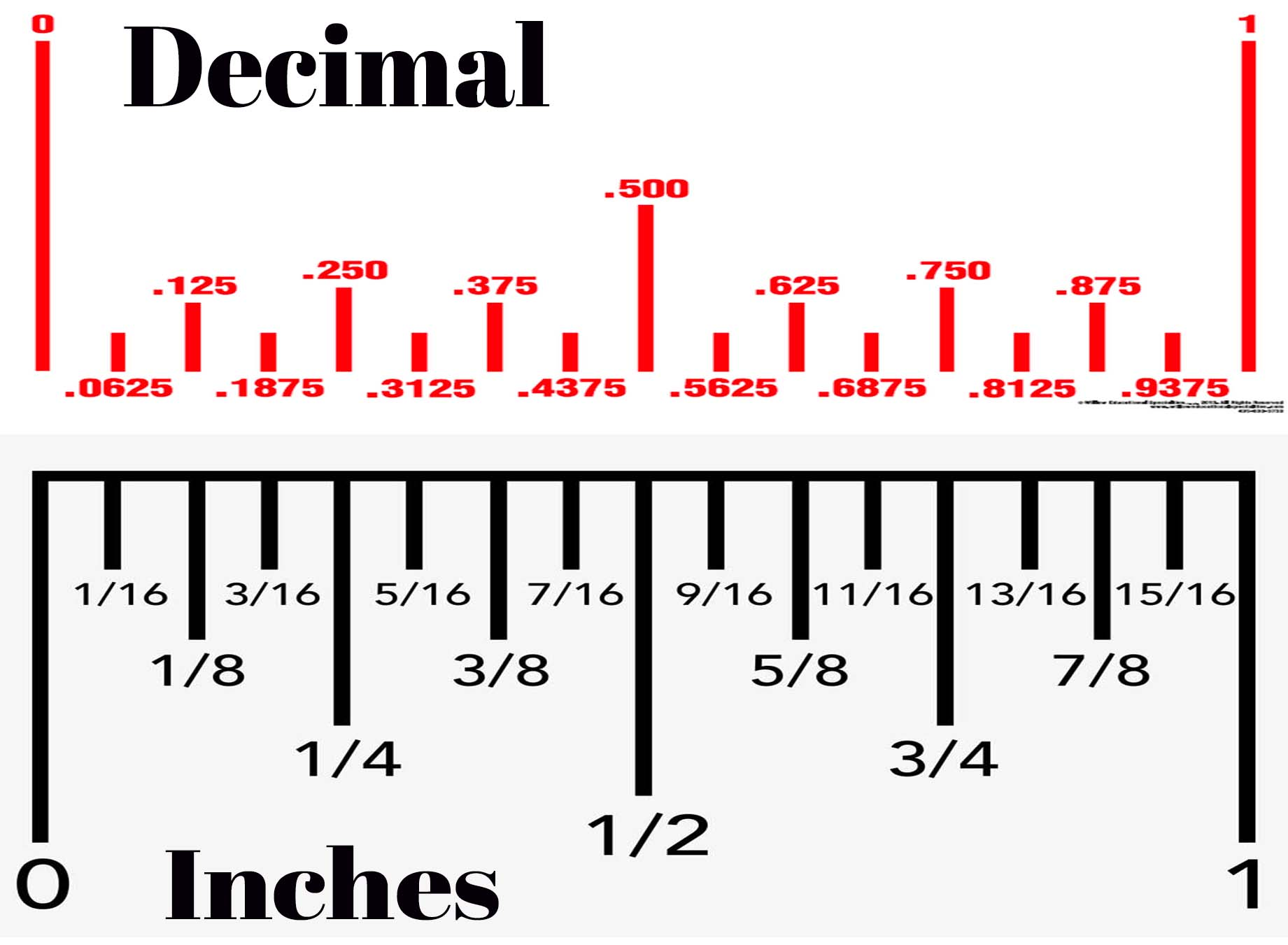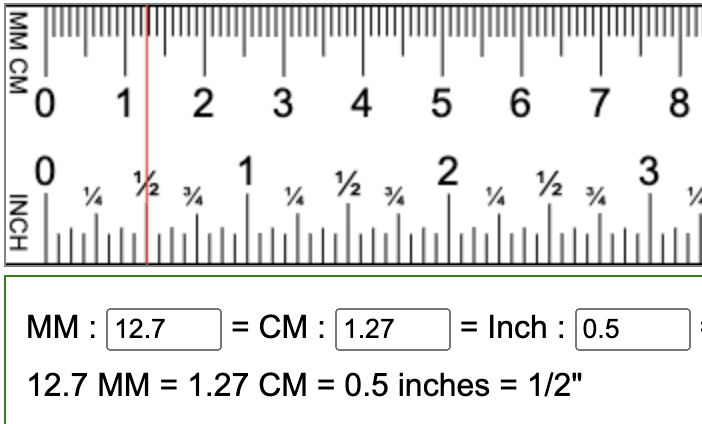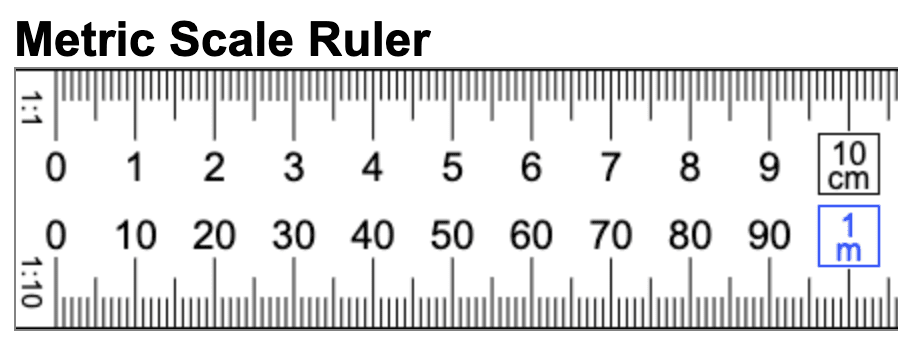How to Convert inches, millimeters, decimals, fractions and more!

# How do I convert measurements? Inches to millimeters, millimeters to centimeters. And fractions to decimals.

One of the most common questions when ordering signs is how do I convert different measurements. Below are some images that should help to explain the difference.

## Here's a detailed explanation for converting between centimeters, millimeters, inches, decimals, and fractions:

1. Converting centimeters to millimeters: To convert centimeters to millimeters, simply multiply the number of centimeters by 10. For example, 5 centimeters is equal to 50 millimeters (5 x 10).

2. Converting millimeters to centimeters: To convert millimeters to centimeters, simply divide the number of millimeters by 10. For example, 50 millimeters is equal to 5 centimeters (50 ÷ 10).

3. Converting inches to centimeters: To convert inches to centimeters, multiply the number of inches by 2.54. For example, 6 inches is equal to 15.24 centimeters (6 x 2.54).

4. Converting centimeters to inches: To convert centimeters to inches, divide the number of centimeters by 2.54. For example, 15.24 centimeters is equal to 6 inches (15.24 ÷ 2.54).

5. Converting decimals to fractions: To convert a decimal to a fraction, write the decimal as a fraction with a denominator of 1. Then multiply both the numerator and denominator by 10, 100, or 1000 to eliminate the decimal point. Finally, simplify the fraction if possible. For example, 0.75 can be written as 75/100, which simplifies to 3/4.

6. Converting fractions to decimals: To convert a fraction to a decimal, divide the numerator by the denominator. For example, 3/4 is equal to 0.75 (3 ÷ 4).

Overall, converting between centimeters, millimeters, inches, decimals, and fractions requires a combination of multiplication, division, and simplification. With practice, you can become more comfortable with these conversions and apply them to a wide range of measurement and mathematical problems.

### Now that you know how to convert measurements, can you explain what each one is?

Centimeters and millimeters are both units of measurement for length, with centimeters being larger than millimeters. There are 10 millimeters in a centimeter.

Inches are also a unit of measurement for length, but they are typically used in countries like the United States, Canada, and the United Kingdom, while centimeters are more commonly used in countries that use the metric system.

Decimals and fractions are different ways of representing numbers. Decimals use a decimal point to separate whole numbers from fractions of a whole, while fractions represent a ratio of two numbers. Fractions are often written using a numerator and denominator, with the numerator representing the number of parts and the denominator representing the total number of parts.

In the context of length measurement, decimals and fractions can be used to represent measurements that are not exact multiples of a given unit. For example, a length of 2.5 centimeters could be represented as either 2 and 1/2 centimeters (a fraction) or 2.5 centimeters (a decimal).

Overall, centimeters, millimeters, inches, decimals, and fractions are all different ways of measuring and representing length. The choice of which unit to use depends on the specific application, with different units being more appropriate in different contexts.Above (or to the left, depending on if you are on a phone, tablet or laptop) is a comparison of Decimals and Inches. The images are lined up with each other so you can see that 1/4" lines up with .250 in decimals. And .500 decimals lines up with 1/2". When ordering different products, you might want a 4mm corplast sign, but in the box where you place your order, you'll see .16Next, the image shows a conversion between Inches, Milimeters and Centimeters:And for those who use the Metric measuring system: